Next: HORIZONTAL REFLECTOR IN A Up: Fomel & Grechka: Nonhyperbolic Previous: Introduction

# WEAK ANISOTROPY APPROXIMATION

In a transversely isotropic medium, velocities of seismic waves depend on the direction of propagation measured from the symmetry axis. Thomsen 1986 has introduced a convenient parametrization of this dependence, replacing the general notation of elastic anisotropy in terms of stiffness coefficients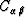by P- and S-wave velocities along the symmetry axis and three dimensionless parameters. As shown by Tsvankin 1996, the P-wave seismic signatures in vertically transverse isotropic (VTI) media can be conveniently expressed in terms of Thomsen's parameters,, and. A deviation of these paramers from zero characterizes the relative strength of anisotropy. Therefore, the weak anisotropy approximation Thomsen (1986); Tsvankin and Thomsen (1994) reduces to simple linearization.

In weakly anisotropic VTI media, the squared group velocity Vg2 of seismic P-waves can be expressed as a function of the group angle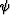as follows: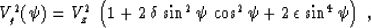(1)
where Vz = Vg(0) is the vertical velocity, andandare Thomsen's dimensionless anisotropic parameters, which are assumed to be small quantities: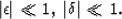(2)
Both parameters are equal to zero in the isotropic case. Their connection with the stiffness coefficients has the following expressions Thomsen (1986):(3) (4)

Equation (1) is accurate up to the second-order terms inand. We retain this level of accuracy throughout this paper without additional clarification. As follows from equation (1), the horizontal velocity Vx corresponding to the horizontal ray propagation is(5)
Equation (5) coincides with the exact expression, which is valid for any strength of anisotropy. Another important quantity is the normal moveout (NMO) velocity for homogeneous VTI media with a horizontal reflector. Its exact expression is Thomsen (1986)(6)

One example of a physical anisotropic model is ANNIE, proposed by Shoenberg, Muir, and Sayers 1996 to describe anisotropy of shales. According to this model, the elasticity tensor (stiffness matrix) in transversely isotropic shales is represented by the three parameter approximation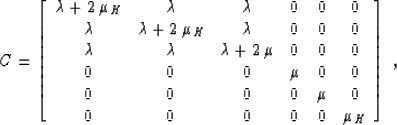(7)
where,, and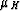are density-normalized elastic parameters. Formula (3) shows that Thomsen's parameterin this case is equal to zero, which corresponds to the known fact that the normal moveout velocity for shales is approximately equal to the vertical velocity. The parameterin this case is defined by the equation(8)

It is convenient to rewrite equation (1) in the form(9)
where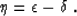(10)
The paramteris equivalent under the weak anisotropy assumption to the anelliptic parameter introduced by Alkhalifah and Tsvankin 1995. For the elliptic anisotropy model,equals, andis equal to zero. To see why the group velocity function becomes elliptic in this case, note that for small,(11)
In practical cases of VTI media,is often greater than, so the anelliptic parameteris positive.

Another equivalent form of equation (1) follows from a substitution of the three characteristic velocities Vz, Vx, and Vn, as follows: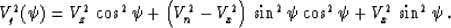(12)
It is apparent in formula (12) that, in the linear approximation, the anelliptic behavior of anisotropy is controlled by the difference between the normal moveout and horizontal velocities.

We illustrate different types of anisotropy in Figure 1, which shows the wavefronts for different values of the anisotropic parameters. The wavefront, circular in the isotropic case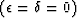, appears elliptic if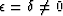. Ifis greater than zero, andis smaller than zero, the three characteristic velocities satisfy the inequality Vx > Vz > Vn.nmofro
Figure 1
Wavefronts in weakly anisotropic media. Solid curves denote anisotropic wavefronts. Dashed curves denote isotropic wavefronts for the corresponding vertical, horizontal, and normal moveout velocities. Top left: isotropic case; top right: elliptic case; bottom left: ANNIE model; bottom right: strongly anelliptic case.Next: HORIZONTAL REFLECTOR IN A Up: Fomel & Grechka: Nonhyperbolic Previous: Introduction
Stanford Exploration Project
11/12/1997